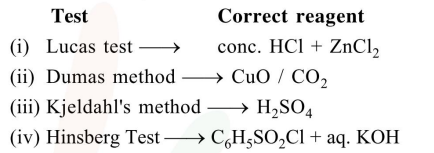# The difference between the radii of

Question:

The difference between the radii of $3^{\text {rd }}$ and $4^{\text {th }}$ orbits of $\mathrm{Li}^{2+}$ is $\Delta \mathrm{R}_{1}$. The difference between the radii of $3^{\text {rd }}$ and $4^{\text {th }}$ orbits of $\mathrm{He}^{+}$is $\Delta \mathrm{R}_{2}$. Ratio $\Delta \mathrm{R}_{1}: \Delta \mathrm{R}_{2}$ is :

1. $8: 3$

2. $3: 2$

3. $3: 8$

4. $2: 3$

Correct Option: , 4

Solution: